•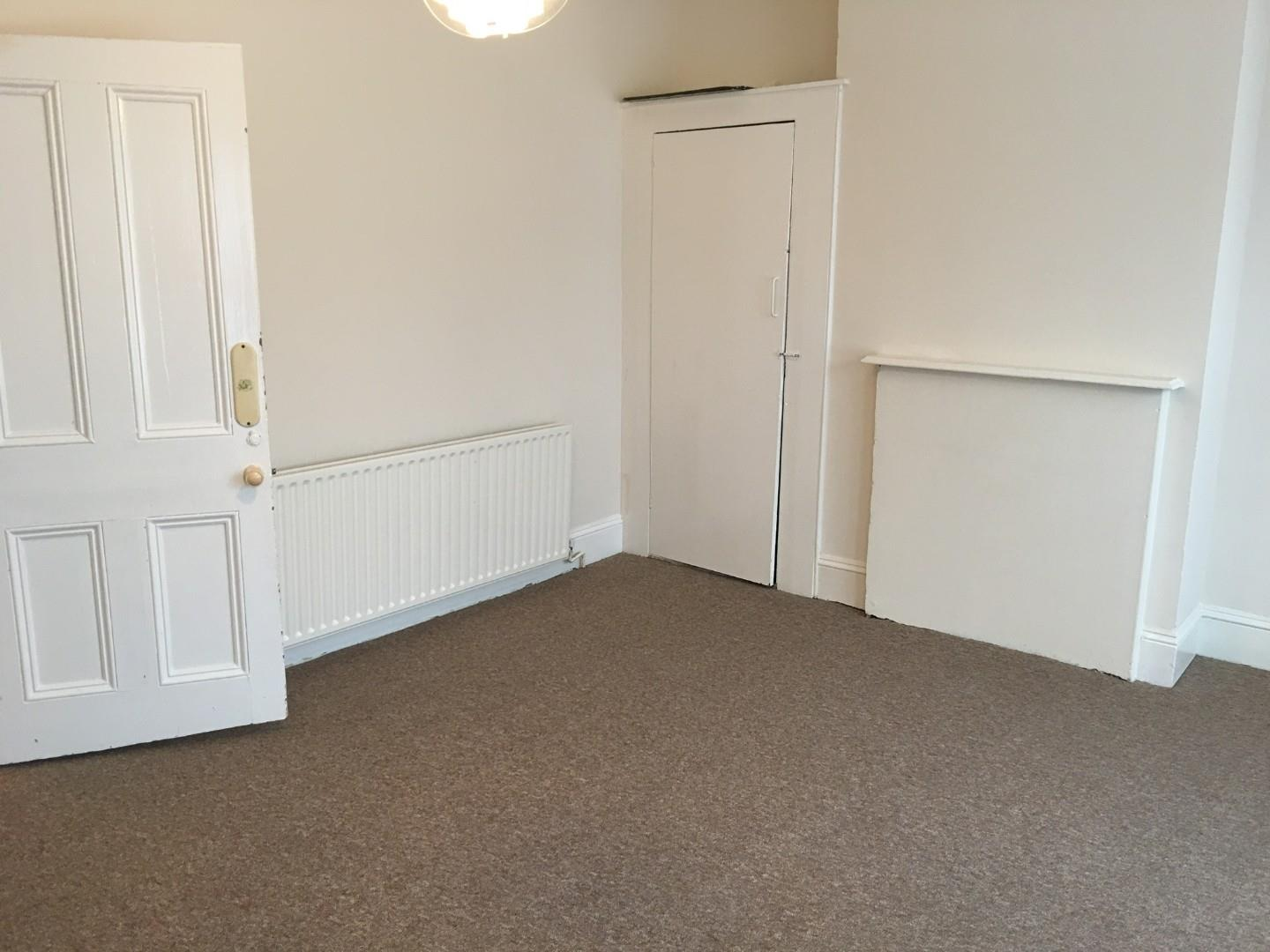£1,350.00 To Let
•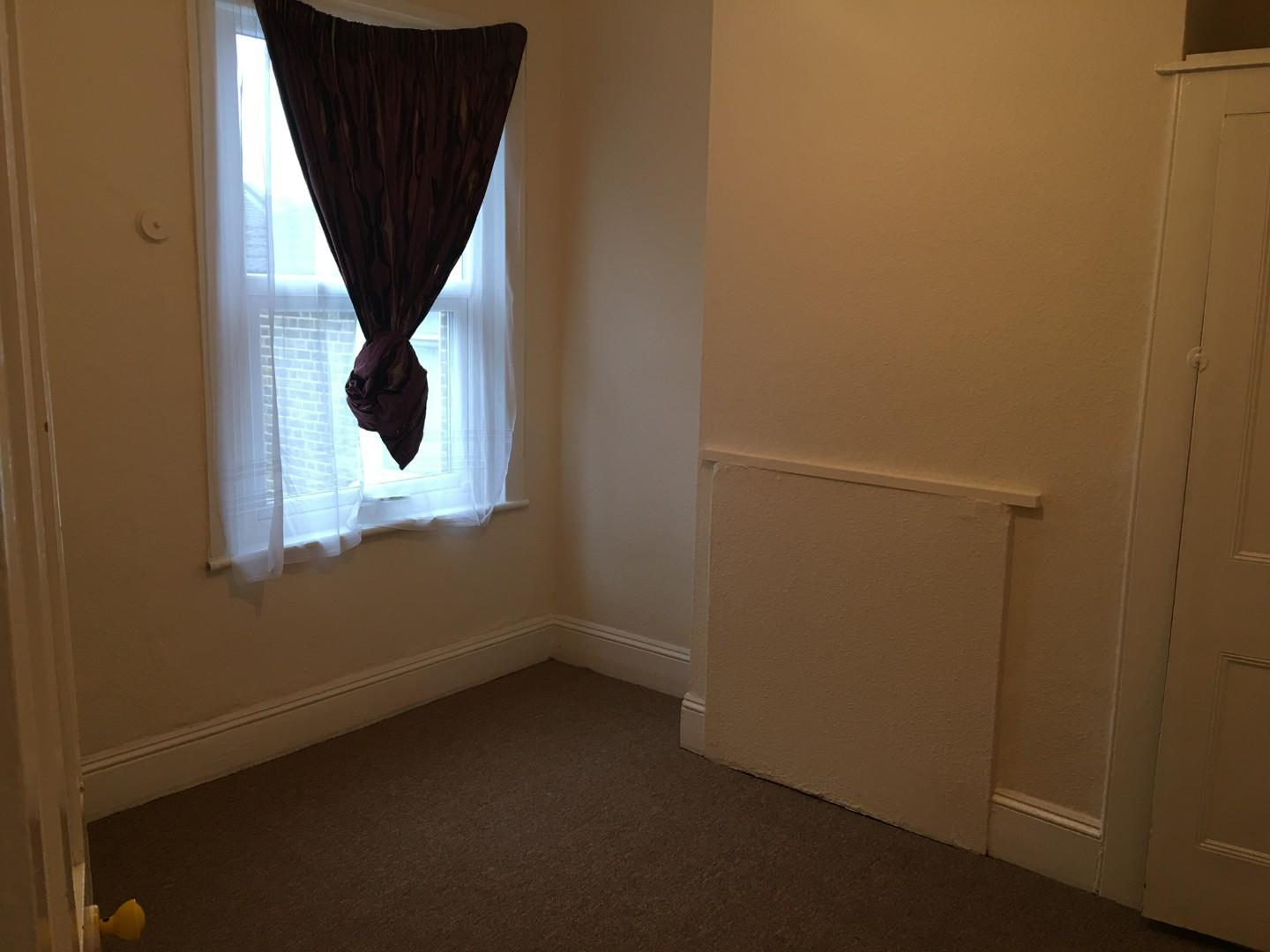£1,350.00 To Let
•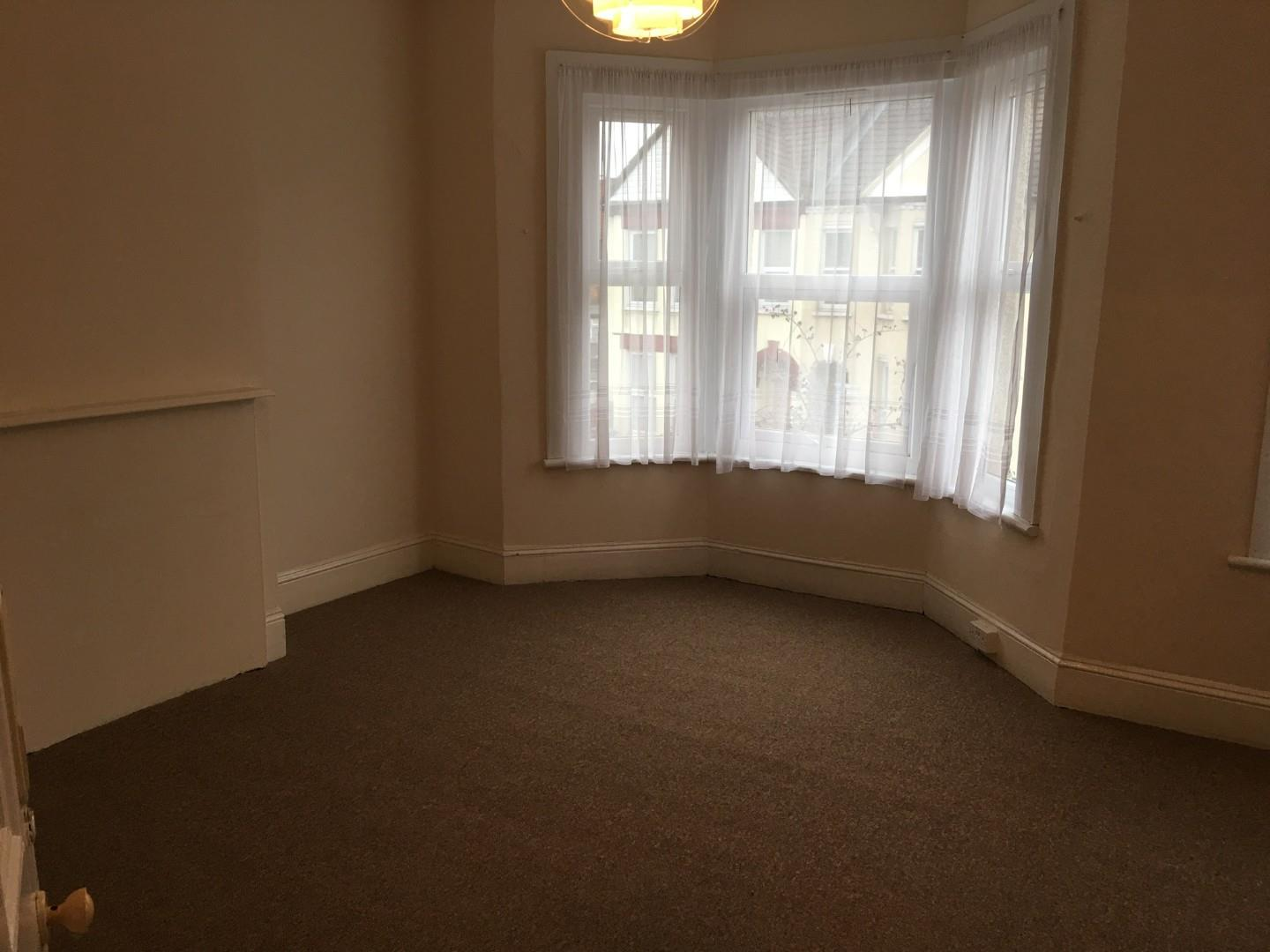£1,350.00 To Let
•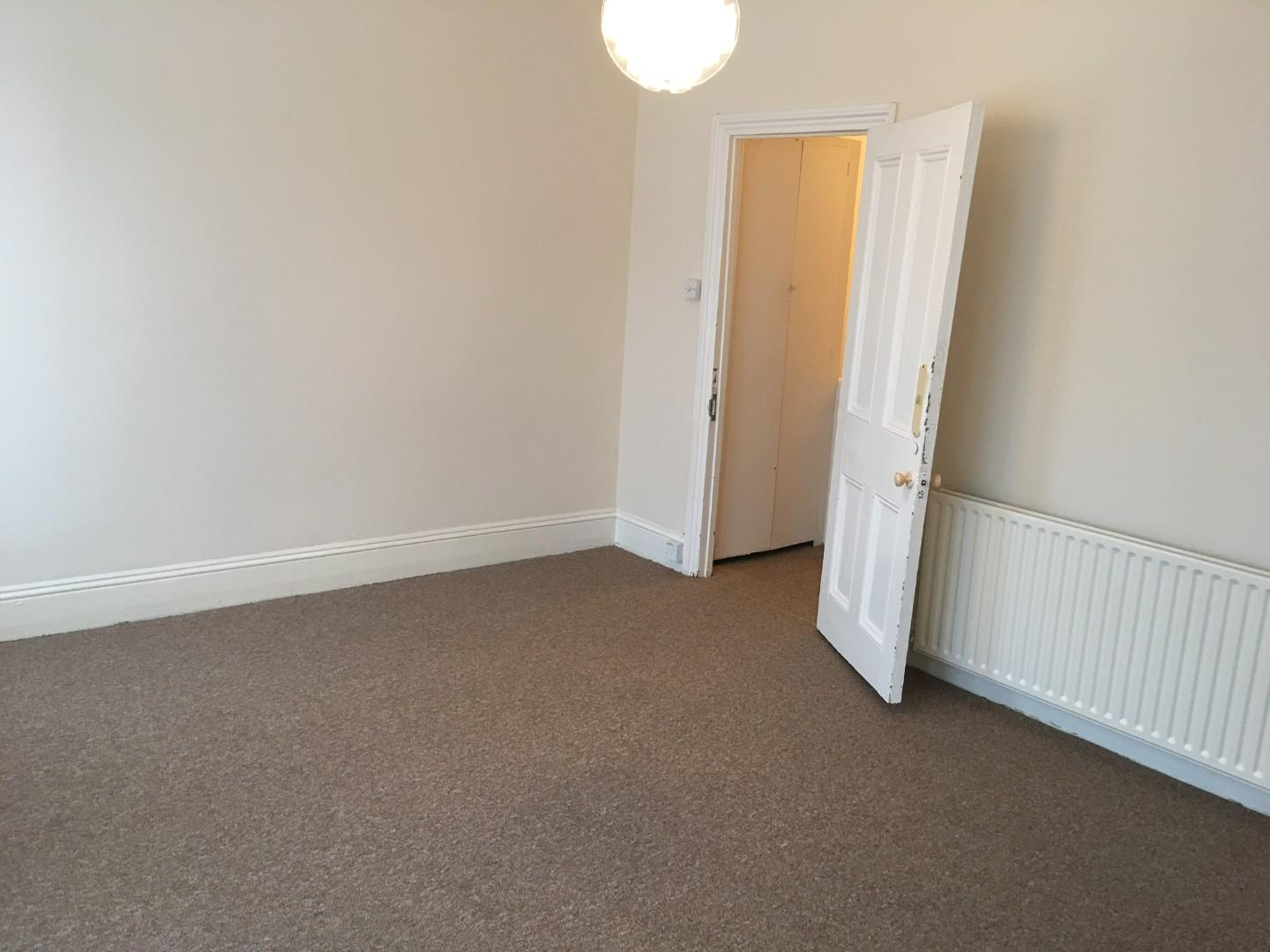£1,350.00 To Let
•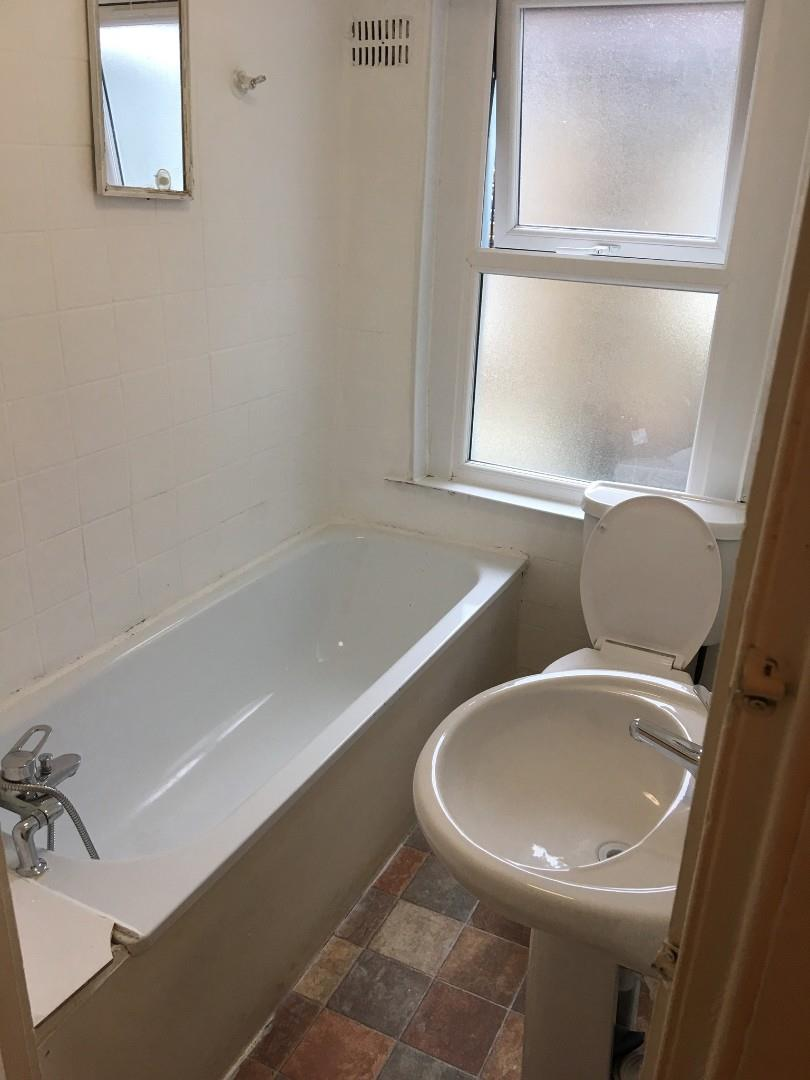£1,350.00 To Let
•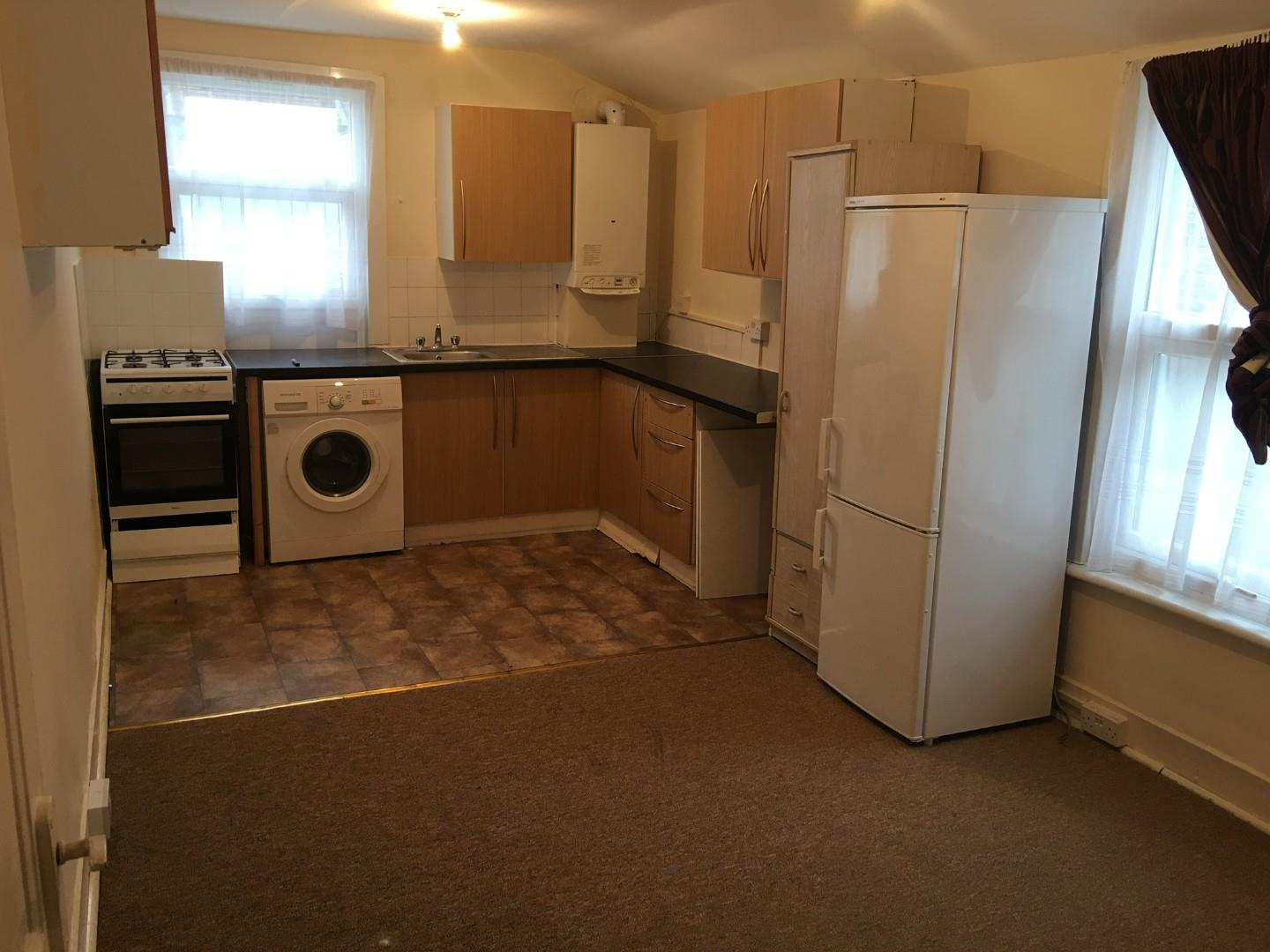£1,350.00 To Let
•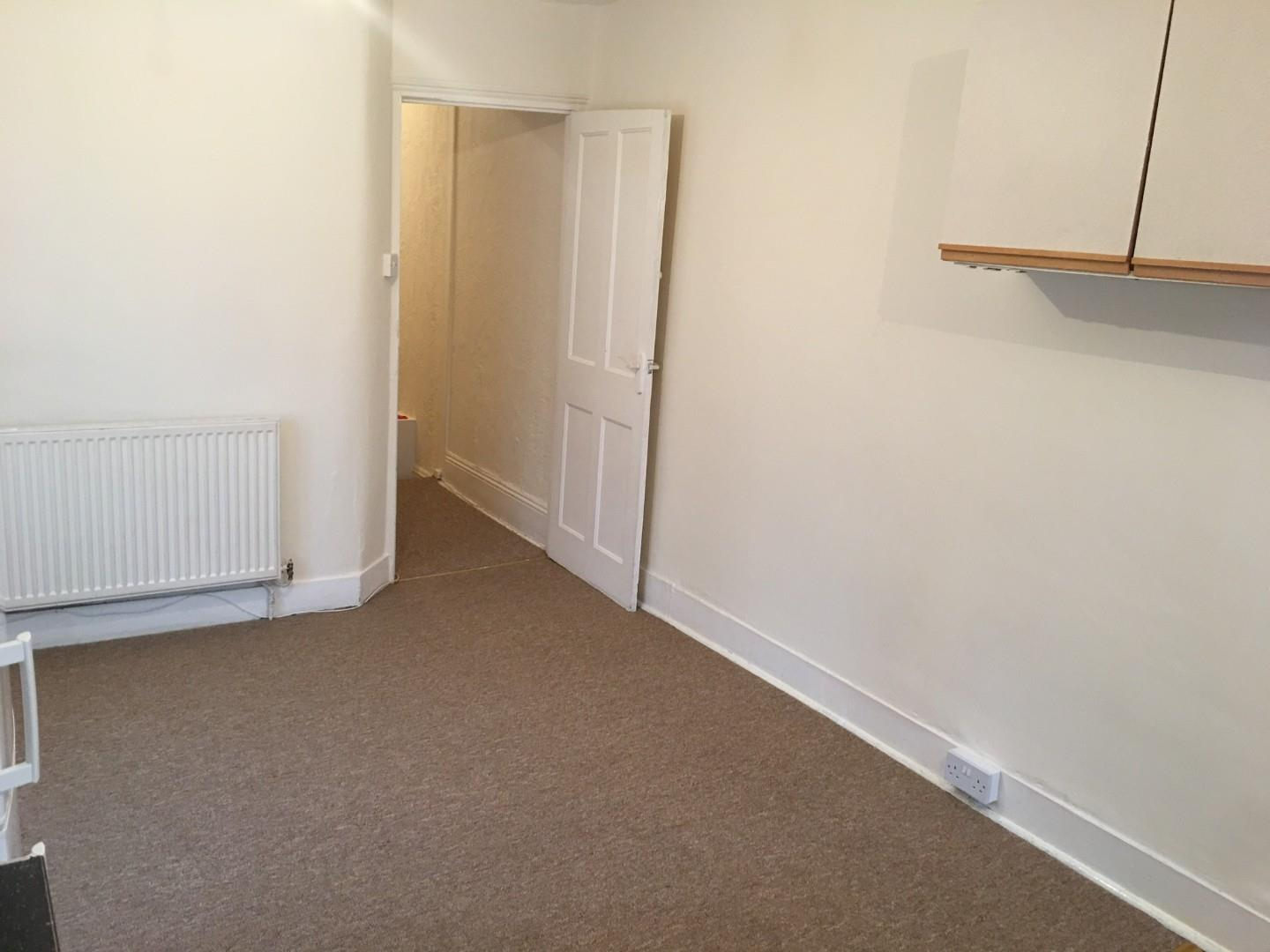£1,350.00 To Let
•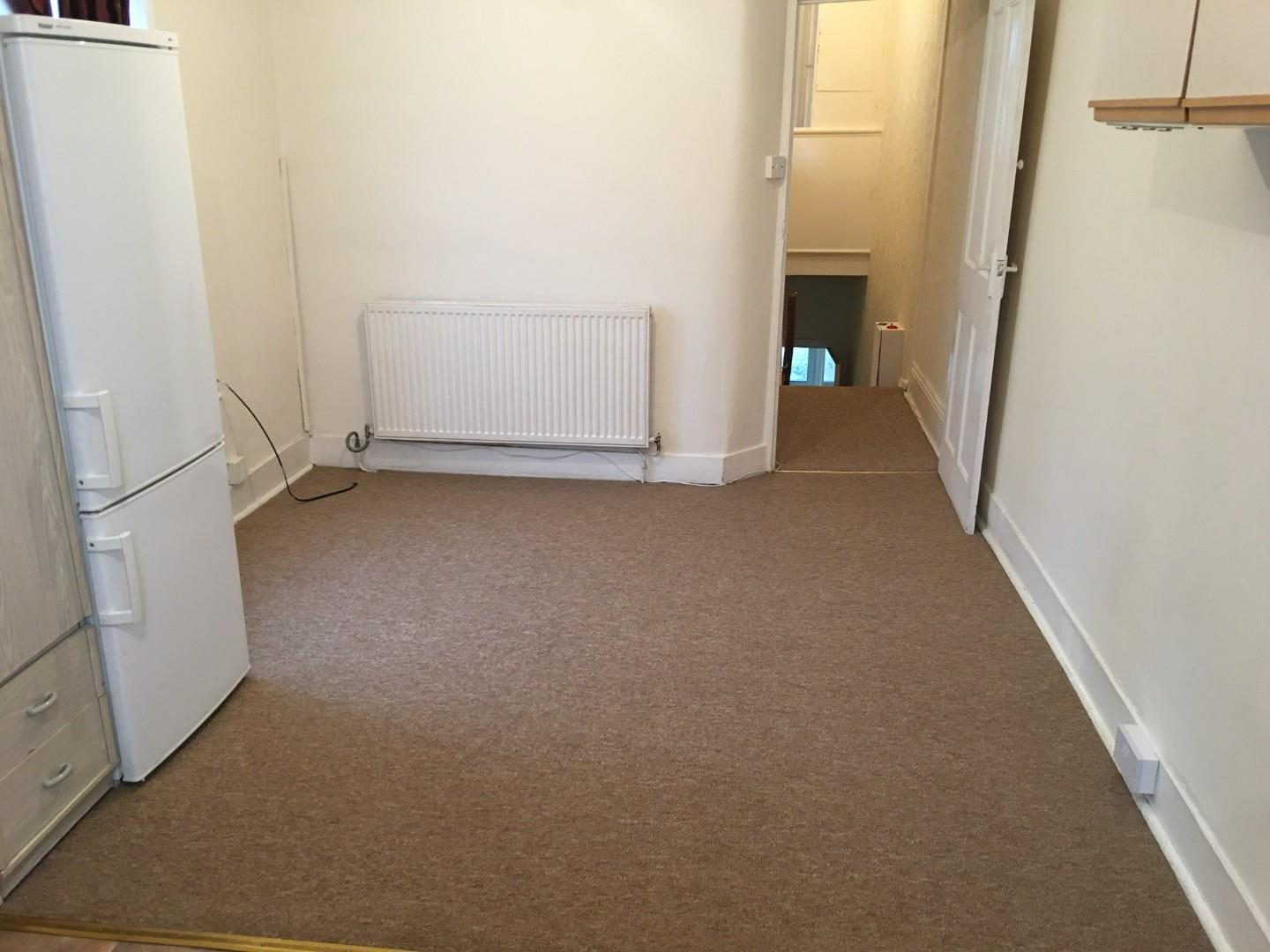£1,350.00 To Let
•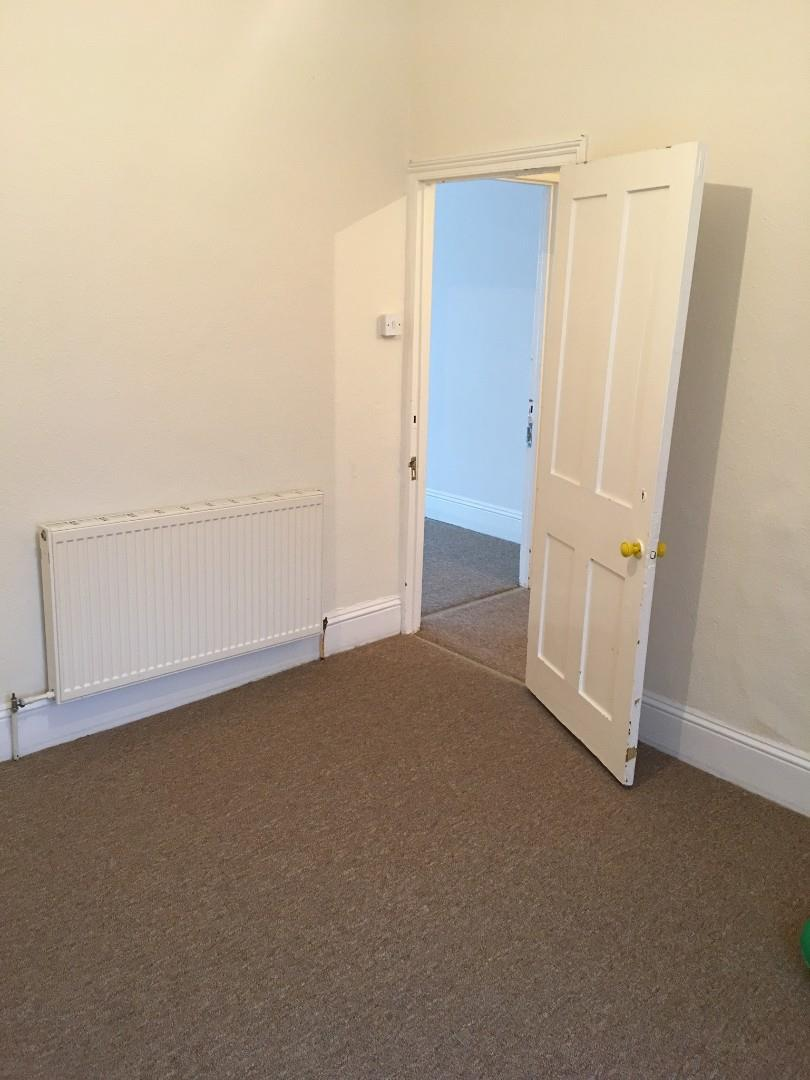£1,350.00 To Let
•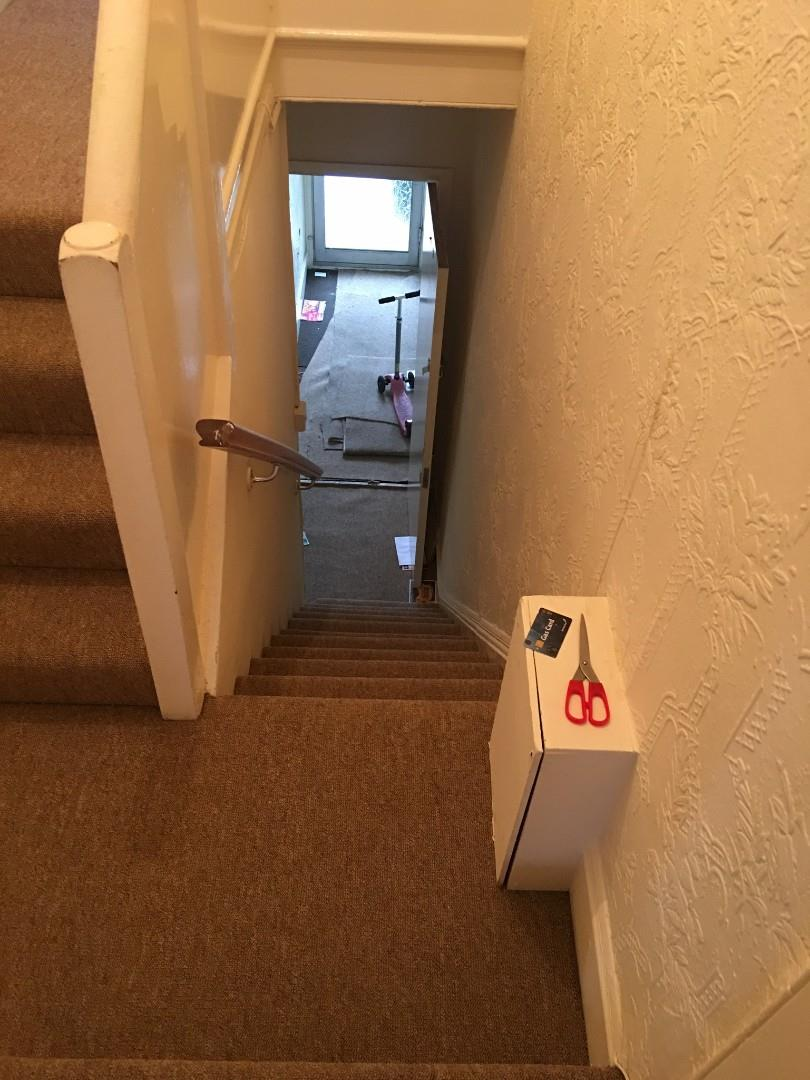£1,350.00 To Let
•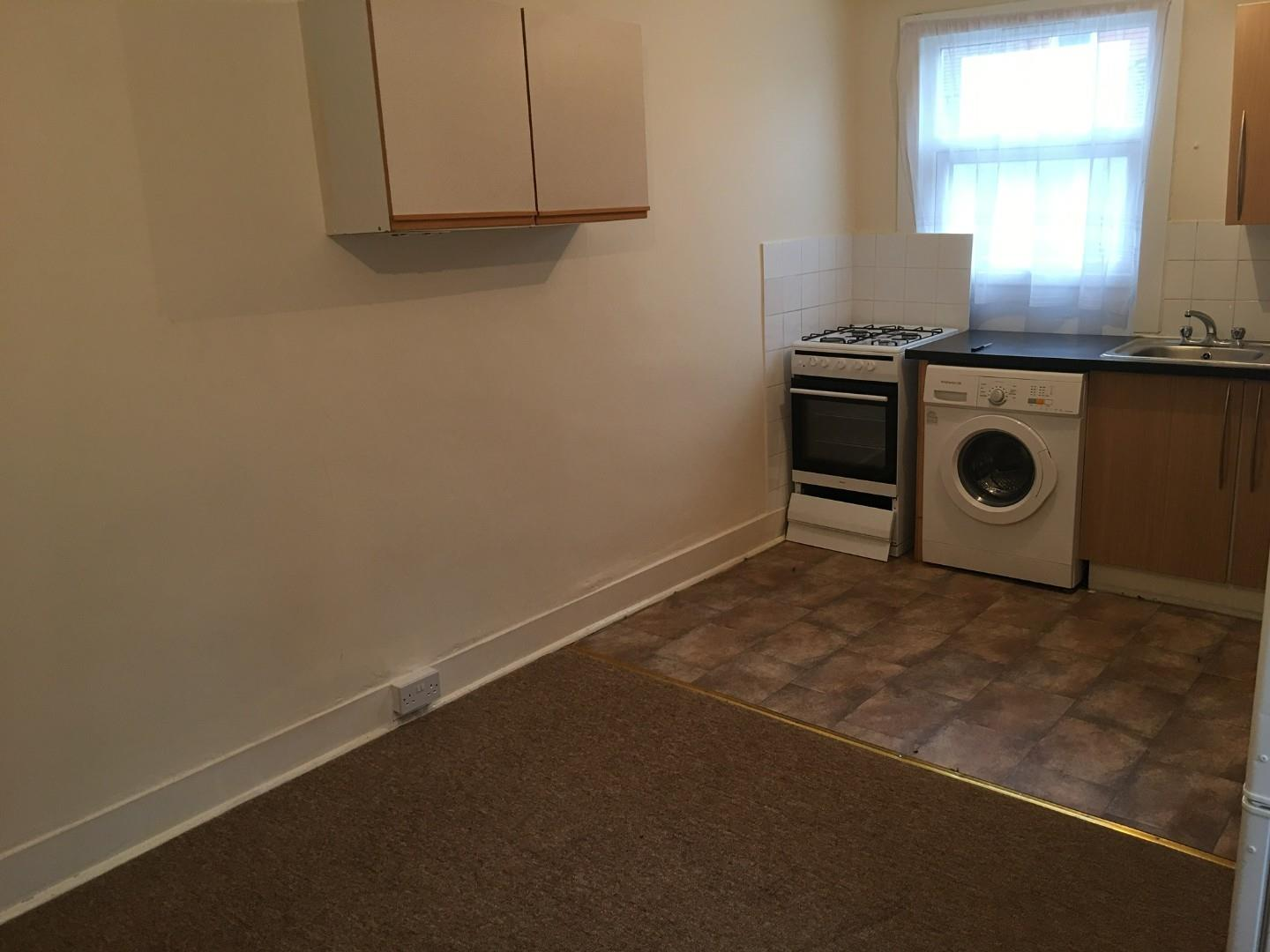£1,350.00 To Let
•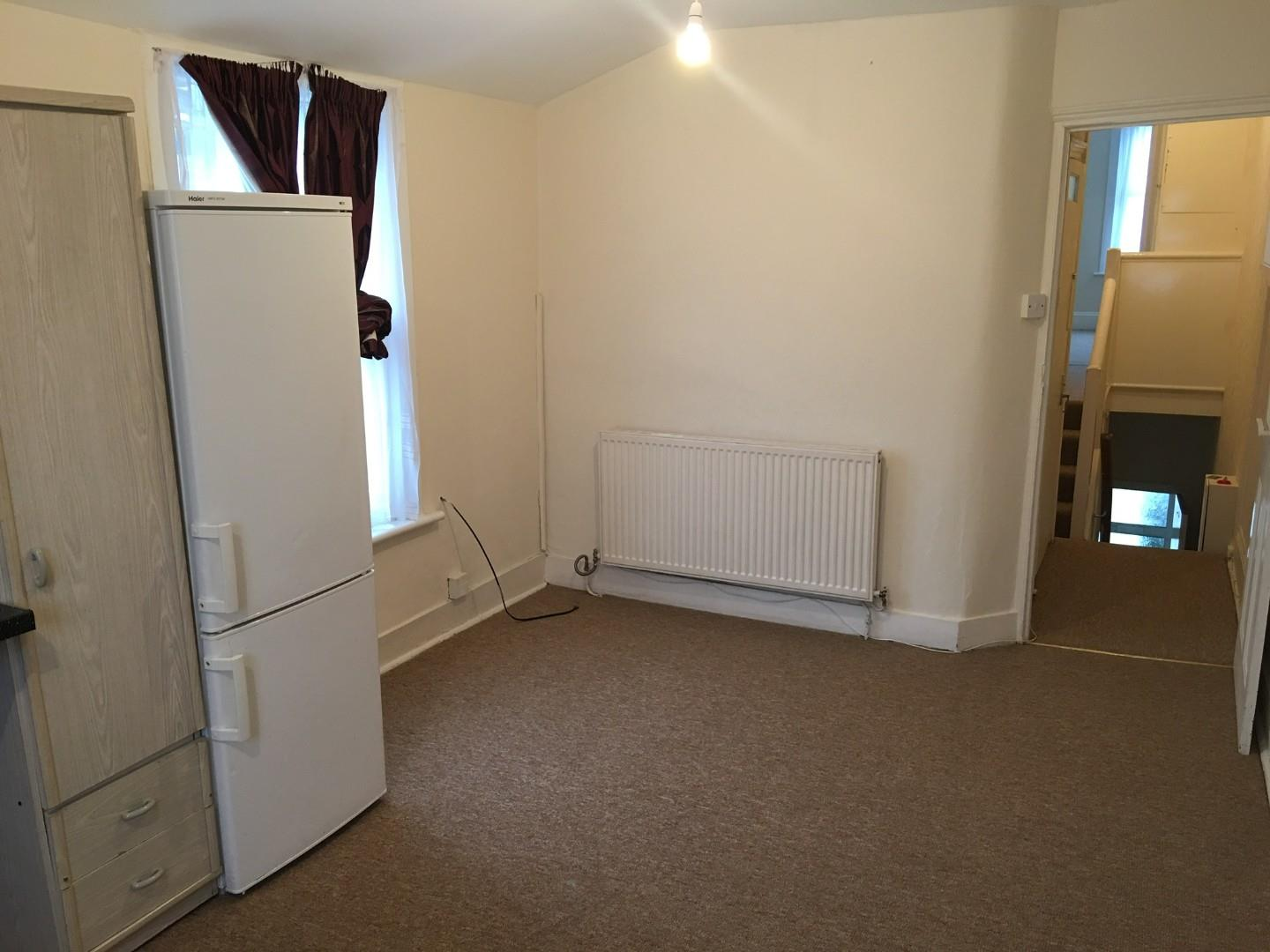£1,350.00 To Let
•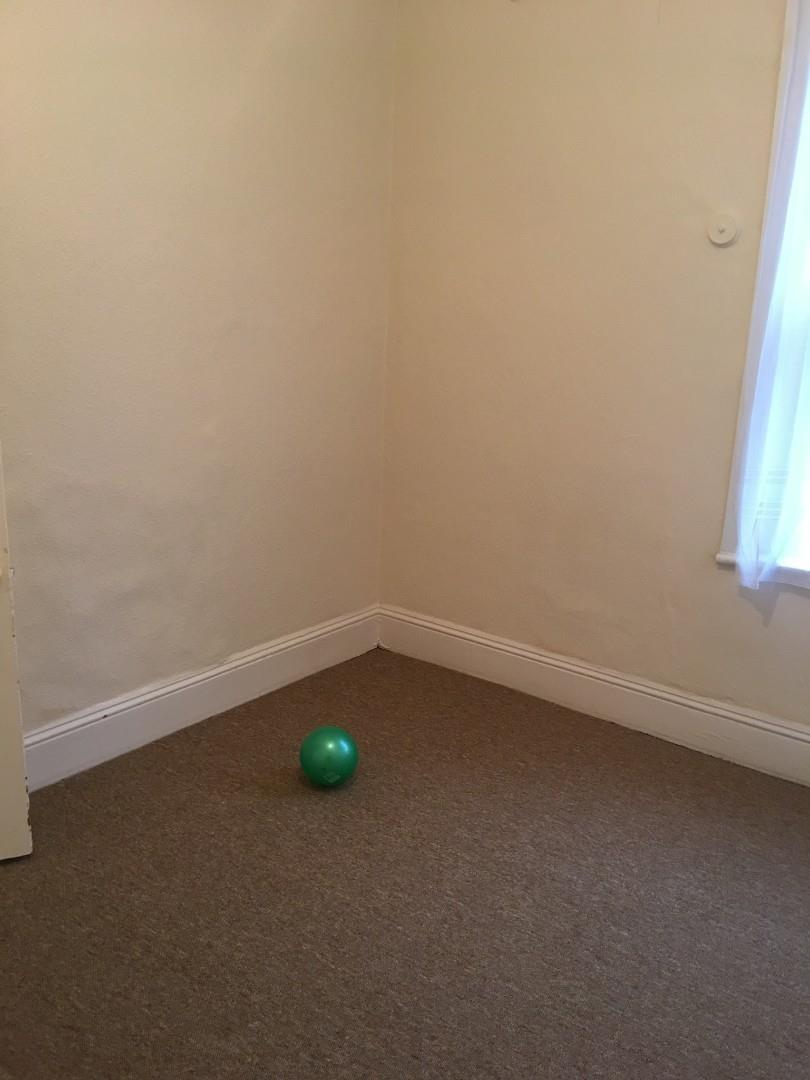£1,350.00 To Let
•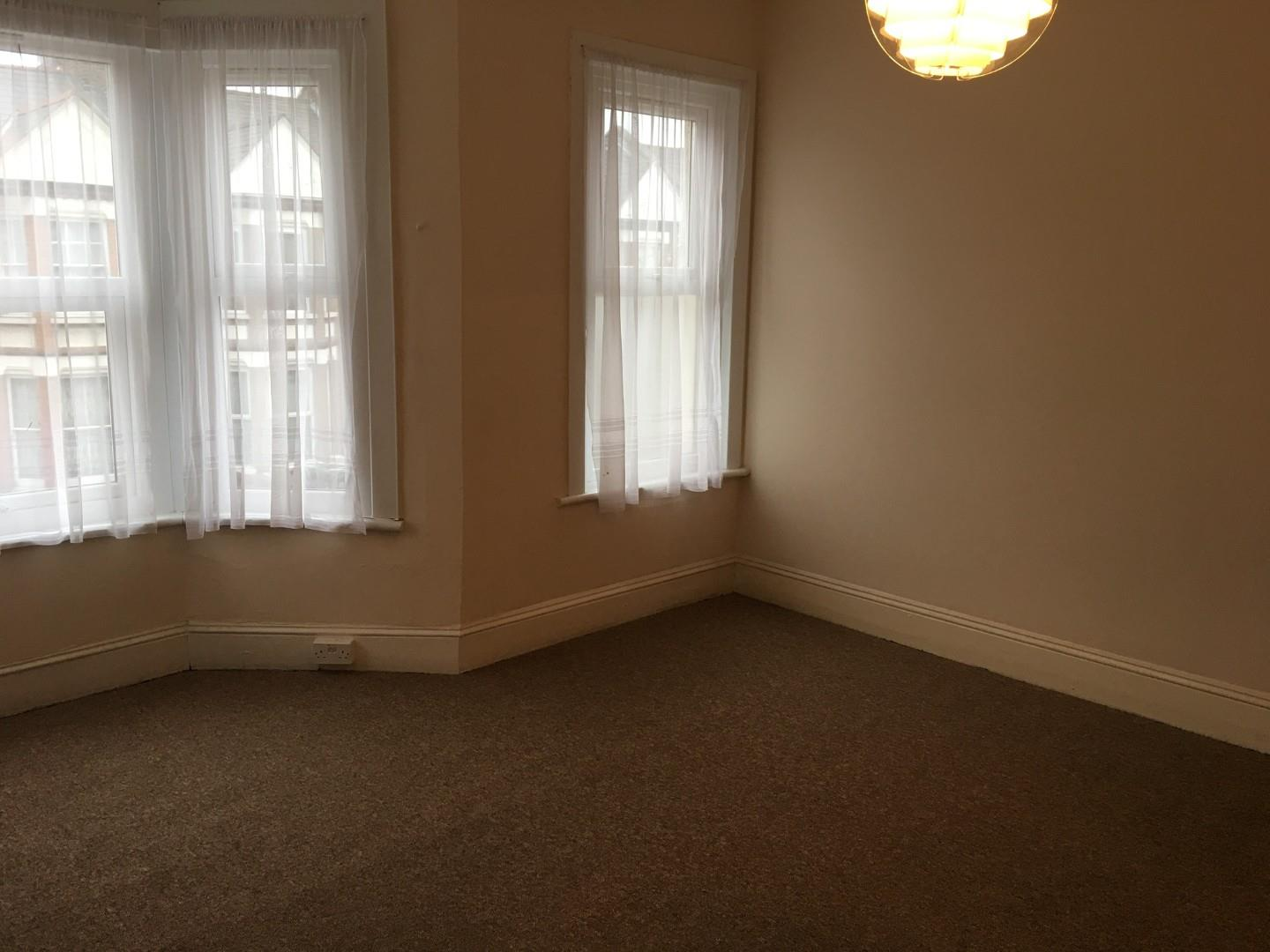£1,350.00 To Let
•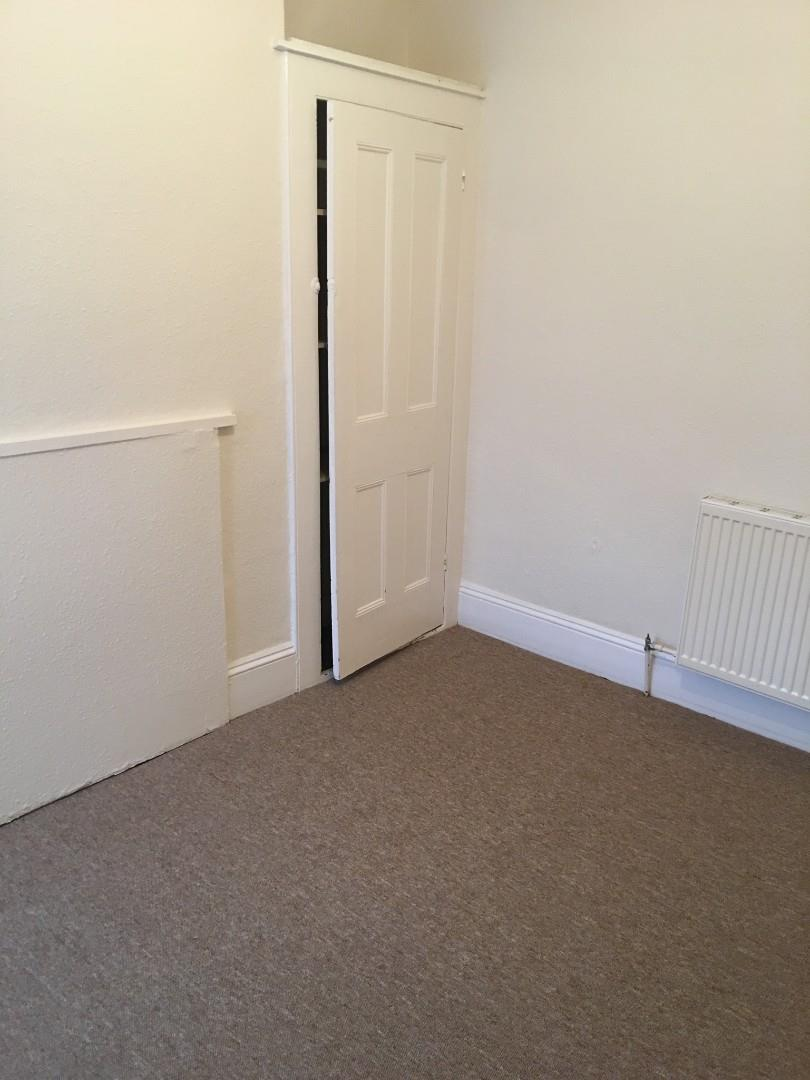£1,350.00 To Let
•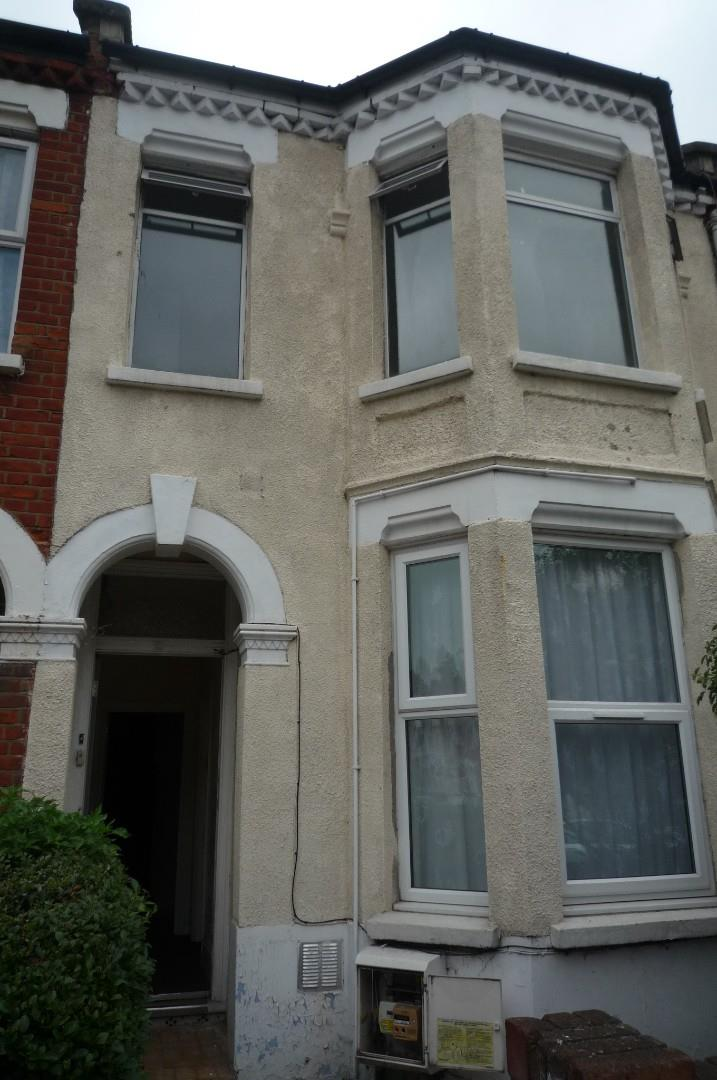£1,350.00 To Let
•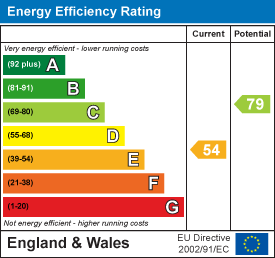£1,350.00 To Let
•£1,350.00 To Let